## Excel Formula: Abbreviate Words or Names

In this tutorial, it provides some formulas to extract initial letters from each word in a cell as below screenshot shown. Also, it explains how the formulas work in Excel.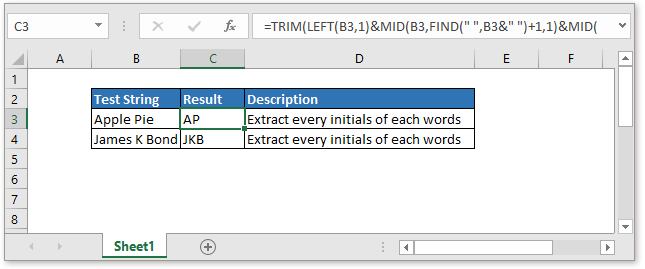### In Office 365 and Excel 2019

The TEXTJOIN function is a new function available in Office 365 and Excel 2019 which can be used to extract all initial letters from each word.

Generic formula:

 TEXTJOIN("",1,IF(ISNUMBER(MATCH(CODE(MID(text,ROW(INDIRECT("1:"&LEN(text))),1)),ROW(INDIRECT("63:90")),0)),MID(text,ROW(INDIRECT("1:"&LEN(text))),1),""))

Arguments

 Text: the text string you want to abbreviate.

How this formula work

Supposing you want to extract initials from cell A1, use this formula in cell B1.

 =TEXTJOIN("",1,IF(ISNUMBER(MATCH(CODE(MID(A1,ROW(INDIRECT("1:"&LEN(A1))),1)),ROW(INDIRECT("63:90")),0)),MID(A1,ROW(INDIRECT("1:"&LEN(A1))),1),""))

Press Shift + Ctrl + Enter key, and all initials of each word will be extracted.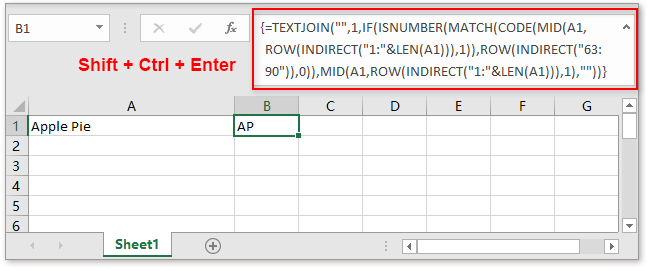Explanation

1. The TEXTJOIN function is used to join text values with delimiter.

2. The MID, ROW, INDIRECT and LEN functions are used to convert the text string to an array or letters. Supposing

 =MID(A1,ROW(INDIRECT("1:"&LEN(A1))),1)

Press Shift + Ctrl + Enter

Returns an array of letters

 A,p,p,l,e,P,i,e

And then this array is fed into CODE function, which outputs an array of numeric ascii codes, one code for each letter.

3. The ROW(INDIRECT("63:90") corresponds to the ascii codes for all capital letters A-Z.

4. The ISNUMBER, IF and MATCH functions are used to filter the results for final text joining.

Remarks:

1. If there are no capital letters in the text string, this formula returns blank.

2. This formula will extract all capital letters.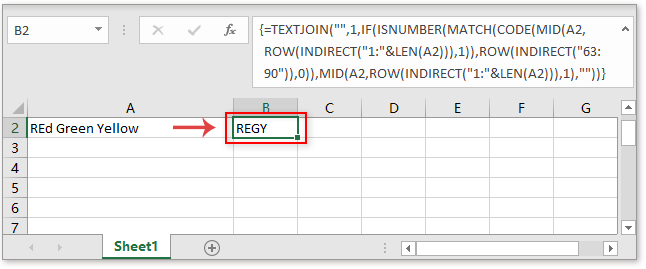### In Office 2016 or previous versions

If you are in Excel 2016 or previous versions, you can use the TRIM function.

Generic formula:

 TRIM(LEFT(Text,1)&MID(Text,FIND(" ",Text&" ")+1,1)&MID(Text,FIND("*",SUBSTITUTE(Text&" "," ","*",2))+1,1))

Arguments

 Text: the text string you want to extract the first letters of each word.

How this formula work

Supposing you want to extract initials from cell A1, use this formula in cell B1.

 =TRIM(LEFT(A1,1)&MID(A1,FIND(" ",A1&" ")+1,1)&MID(A1,FIND("*",SUBSTITUTE(A1&" "," ","*",2))+1,1))

Press Enter key, all first letters of each word in cell A1 is extracted.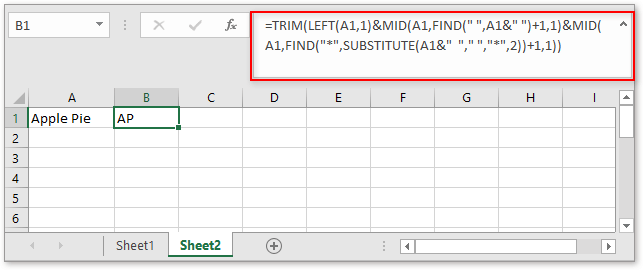Explanation

1. The TRIM function removes all extra spaces in the text string.

2. The LEFT(A1,1) extracts the first letter of the text string.

3. MID(A1,FIND(" ",A1&" ")+1,1) extracts the first letter of the second word which is separated by space.

4. MID(A1,FIND("*",SUBSTITUTE(A1&" "," ","*",2))+1,1)) extracts the first letter of the third word which is separated by space.

Remarks:

1. This formula only works for three or less words in a cell.

2. You can change “ “ in the formula to other delimiters.

3. This formula extracts the first letters in case insensitive, if you want the formula always returns in upper case, add the UPPER function to the formula

 =UPPER(TRIM(LEFT(A1,1)&MID(A1,FIND(" ",A1&" ")+1,1)&MID(A1,FIND("*",SUBSTITUTE(A1&" "," ","*",2))+1,1)))

### Sample File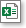Click to download sample file

### The Best Office Productivity Tools

#### Kutools for Excel - Helps You To Stand Out From Crowd

 Popular Features: Find, Highlight or Identify Duplicates  |  Delete Blank Rows  |  Combine Columns or Cells without Losing Data  |  Round without Formula ... Super VLookup: Multiple Criteria  |  Multiple Value  |  Across Multi-Sheets  |  Fuzzy Lookup... Adv. Drop-down List: Easy Drop Down List  |  Dependent Drop Down List  |  Multi-select Drop Down List... Column Manager: Add a Specific Number of Columns  |  Move Columns  |  Toggle Visibility Status of Hidden Columns  |  Compare Columns to Select Same & Different Cells ... Featured Features: Grid Focus  |  Design View  |  Big Formula Bar  |  Workbook & Sheet Manager | Resource Library (Auto Text)  |  Date Picker  |  Combine Worksheets  |  Encrypt/Decrypt Cells  |  Send Emails by List  |  Super Filter  |  Special Filter (filter bold/italic/strikethrough...) ... Top 15 Toolsets:  12 Text Tools (Add Text, Remove Characters ...)  |  50+ Chart Types (Gantt Chart ...)  |  40+ Practical Formulas (Calculate age based on birthday ...)  |  19 Insertion Tools (Insert QR Code, Insert Picture from Path ...)  |  12 Conversion Tools (Numbers to Words, Currency Conversion ...)  |  7 Merge & Split Tools (Advanced Combine Rows, Split Excel Cells ...)  |  ... and more

Kutools for Excel Boasts Over 300 Features, Ensuring That What You Need is Just A Click Away...#### Office Tab - Enable Tabbed Reading and Editing in Microsoft Office (include Excel)

• One second to switch between dozens of open documents!
• Reduce hundreds of mouse clicks for you every day, say goodbye to mouse hand.
• Increases your productivity by 50% when viewing and editing multiple documents.
• Brings Efficient Tabs to Office (include Excel), Just Like Chrome, Edge and Firefox.